﻿ 星形连接 (Y)：三相电源、电压和电流值介绍-苏州吉事励电源厂家
ENGLISH
ENGLISH

什么是星形连接 (Y)? 　　星形连接(Y)系统也称为三相四线制(3-Phase 4 Wire)，是交流配电的首选系统，而传输时通常使用三角形连接。

什么是星形连接 (Y)?

星形连接(Y)系统也称为三相四线制(3-Phase 4 Wire)，是交流配电的首选系统，而传输时通常使用三角形连接。

在星形(也用Y表示)互连系统中，三个线圈的始端或终点(相似端)连接在一起形成中性点。

星形连接是通过将三个线圈的相似端连接在一起获得的，“开始”或“结束”。另一端与线路连接。公共点称为中性点或星点，用N表示。(如图1所示)

星形连接也称为三相四线(3-Phase, 4-Wires)系统。

如果平衡对称负载并联连接在三相电压系统上，那么三个电流将流过中性线，它们的数量相同，但它们相差 120°(异相)，因此这些电流的矢量和三电流 = 0. 即

I R + I Y + I B = 0 ……………。维多利亚时代

任意两个端子之间的电压或线与中性线(星点)之间的电压称为相电压 或星形电压，用V Ph表示。两条线之间的电压称为线间电压或线电压，用V L表示。星形连接 (Y) 中的电压、电流和功率值

现在我们将找到三相星形交流系统中线电流、线电压、相电流、相电压和功率的值。

星形连接中的线电压和相电压

我们知道线 1 和线 2 之间的线电压(从图 3a)是

V RY = V R – V Y .... (矢量差)

因此，要找到 V RY的向量，在反方向增加 V Y的向量，如下图 2 中的虚线所示。同样，在向量 V R和向量 V Y 的两端，做垂直的虚线看起来像一个平行四边形，如图(2)所示。将平行四边形分成两部分的对角线，显示 V RY的值。V Y和 V之间的角度 R矢量是 60°。

因此，如果

V R = V Y = V B = V PH

然后

V RY = 2 x V PH x Cos (60°/2)

= 2 x V PH x Cos 30°

= 2 x V PH x (√3/2) …… 因为 Cos 30° = √3/2

V RY = √3 V PH

相似地，

V YB = V Y – V B

V YB = √3 V PH

和

V BR = V B – V R

V BR =√3V PH

因此，证明V RY = V YB = V BR是星形连接的线电压(V L)，因此，星形连接;

V L = √3 V PH或 V L = √3 E PH

星形连接中的线电压和相电压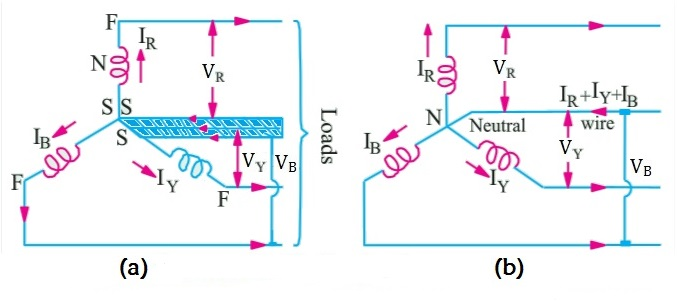从图2中可以看出;

线电压彼此相距 120°

线电压与相应的相电压相差 30°

线电流与相应线电压的夹角Ф为(30°+Ф)，即每条线电流都滞后(30°+Ф)相应的线电压。

星形连接中的线电流和相电流

从图(3a)可以看出，每条线都与单独的相绕组串联，因此，线电流值与线所连接的相绕组中的电流值相同。IE;

线路 1 中的电流 = I R

线路 2 中的电流 = I Y

线路 3 中的电流 = I B

由于三条线路中流动的电流相同，每条线路中的单个电流等于相应的相电流，因此;

I R = I Y = I B = I PH .... 相电流

线电流 = 相电流

I L = I PH

简单来说，线电流和相电流的值在星形连接中是相同的。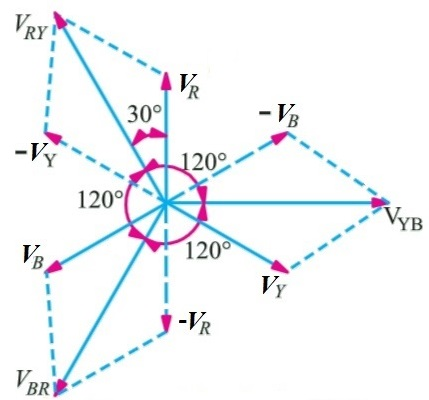星形连接 (Y)：线电流和电压值以及相电流和电压值

星形连接电源

在三相交流电源电路中，总有功或有功功率是三相功率之和。或者所有三相功率的总和是总有功功率或有功功率。

因此，三相交流系统中的总有功或有功功率;

总真功率或有功功率 = 3 相功率

或者

P = 3 x V PH x I PH x CosФ ..... Eq ... (1)

我们知道星形连接的相电流和相电压值;

I L = I PH

V PH = V L /√3 .....(来自V L = √3 V PH)

将这些值放在功率方程中...... (1)

P = 3 x (V L /√3) x I L x CosФ …….. (V PH = V L /√3)

P = √3 x√3 x (V L /√3) x I L x CosФ ..... {3 = √3x√3}

P = √3 x V L x I L x CosФ

因此证明;

星形连接电源，

P = 3 x V PH x I PH x CosФ 或

P = √3 x V L x I L x CosФ

同样的解释在 3-Phase Circuit MCQs with anexplanatory Answer (MCQs No.1) 中也有同样的解释

相似地，

总无功功率 = Q = √3 x V L x I L x SinФ

其中Cos Φ = 功率因数= 相电压和相电流之间的相角，而不是线电流和线电压之间的相角。

很高兴知道：感应线圈的无功功率被视为正极 (+)，电容器的无功功率被视为负极 (-)。

此外，三相的总视在功率;

总视在功率 = S = √3 x V L x I L或，

S = √ (P 2 + Q 2 )。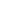0512-68319849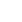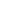top